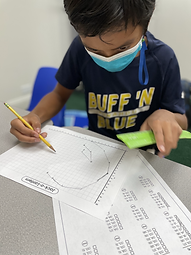## Ms. Mari

### Target 1​

###### Lesson Type:

Continuation

Algebra

:

Variable

Solve equations that use variables to represent unknown numbers.

###### 1:

Identify the unknown information in problems.

3rd

###### Vocabulary:

Variable, Missing Number

Activities:

• used problem solving skills to determine which equation they should solve for first
• determined the value of the three halloween pictures presented to make all equations make sense### Home Exploration

###### Challenge Problem:

Find the values of each shape!

###### Guiding Questions:
• Which equation should you solve for first?## Absent Students:

### Target 2

:

###### 1:

Understand that an exponent indicates the number of times that a base is multiplied by itself.

###### 2:

Write a repeating multiplication equation that is equivalent to a given exponent.

4th

###### Vocabulary:

Exponent, Repeat, Multiplication

Activities:

• flipped two cards (the first card is the base number and the second card is the exponent)
• used what they know about multiplication to solve for the exponent presented
• used PEMDAS to solve multi-step problems that included exponents### Home Exploration

###### Guiding Questions:### Target 3

:

4th

###### Vocabulary:

Coordinates, X-axis, Y-axis

Activities:

• used the coordinate pairs in each section and plotted them on a coordinate grid
• connected the lines for section of coordinate pairs
• created a picture of a jack-o-lantern after connecting all the coordinates together### Home Exploration